# Water pool

Pool with volume 990hl completely filled, if water flows by one tap 8 hours and by second tap 6 hours. First tap give 10hl more than second per hour. How many hl flows in each of them in an hour?

a =  75 hl/h
b =  65 hl/h

### Step-by-step explanation:

990 = 8 a + 6b
a = 10 + b

990 = 8•a + 6•b
a = 10 + b

8a+6b = 990
a-b = 10

a = 75
b = 65

Our linear equations calculator calculates it.Did you find an error or inaccuracy? Feel free to write us. Thank you!Tips to related online calculators
Do you have a linear equation or system of equations and looking for its solution? Or do you have a quadratic equation?
Tip: Our volume units converter will help you with the conversion of volume units.
Do you want to convert time units like minutes to seconds?

## Related math problems and questions:

• Tributaries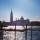The first tributary fill pool with water in 15 hours. The second tributary fill pool in 10 hours. For how many hours the pool is filled with both tributaries?
• Pool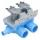Pool is filled with two water supply. The first supply fill pool for nine hours, the second for six hours. How many hours will take fill the pool when the water flows in through the first supply 3 hours and then we will open a second supply?
• Two water containers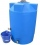In the first container, there are 200 m3 of water and in the second 40 m3. The first container will flow down at a rate of 10 m3 water per hour. At the same time flows to the second rate of 5 m3 per hour. After how many hours there will be three times les
• Pool 2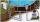The first supply by the pool fill for five hours and the second fill for six hours, drain should be drained for 15 hours. For how many hours the pool is full, when we open both inlet now and outlet open two hours later?
• The dam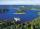The water reservoir is filled with first tributary for 25 hours second tributary for 35 hours. Some time were both inlets open, then a second tributary closed and the tank was filled in four hours. What time water flowed from both tributaries? (Expressed
• Water inlets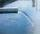Inlet valve with a flow rate of 12 liters per second is filled tank for 72 minutes. How long take to fill full tank if we open one more such valve half an hour after?
• 4 pipes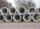The tank flows out by 4 pipes in 6 hours 120 hl water. How much water flows out of 5 pipes of the same diameter in 14 hours?
• Pumps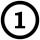First pump flows 16 liters per second into the basin by the second pump 75% of the first and by third pump half more than the second. How long will take fill basin by all three pumps simultaneously volume 15 m3 (cubic meters)?
• The pool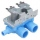The pool contains 220 m3 of water. The pool can be emptied either: a) 10 hours of pipe B and 8 hours of pipe A, or b) 10 hours of pipe A and 7 hours of pipe B. How many cubic meters of water will flow in 1 hour from pipe A and how many from pipe B?
• Tank 9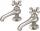The tank with volume V is filled with one pump for three hours and by the second pump for 5 hours. When both pumps run simultaneously, calculate: a) how much of the total volume of the tank is filled in one hour b) for how long is the tank full
• PoolIf water flows into the pool by two inlets, fill the whole for 19 hours. The first inlet filled pool 5 hour longer than the second. How long pool take to fill with two inlets separately?
• Tank No 8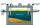Tank is filled by one inlet valve with a flow rate of 12 liters per second in 72 minutes. How long take the tank to fill, if we open half an hour after one more inlet?
• Apprentice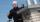Worker and apprentice execute the work for 6 hours. The worker performs himself in 10 hours. How long do the work of an apprentice?
• Two workers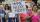The first worker completed the task by himself in 9 hours, the second in 15 hours. After two hours of joint work left first worker to a doctor and the second finished the job himself. How many hours worked second worker himself?
• Tributaries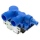We can fill the pool with two different tributaries. The first inflow would fill the pool in 18 hours, both in 6 hours. How many hours would the pool fill with a second inflow?
• Two tributaries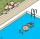Two tributaries of the pool fill it in 10 hours. One of the tributaries would fill 15 hours. How long would fill the first tributary?
• Two pipesHow long will the pool be filled with a double supply pipe if it takes the pool to fill the first pipe by 4 hours longer and the second pipe 9 hours longer than both pipes open at the same time?